Get instant live expert help with Excel or Google Sheets“My Excelchat expert helped me in less than 20 minutes, saving me what would have been 5 hours of work!”

#### Post your problem and you’ll get Expert help in seconds.

Your message must be at least 40 characters
Our professional Expert are available now. Your privacy is guaranteed.

# Sum if cell contains text in another cell

When we want to add data based on a given criteria in Excel, it is best to use SUMIF or SUMIFS.  This article will explain how to sum cells while looking up specific text in another cell.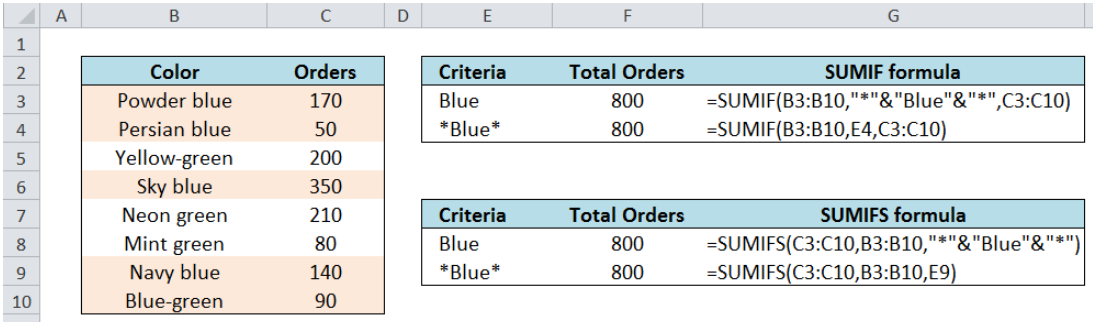Figure 1.  Final result: Sum if cell contains text in another cell

Formula using SUMIF: `=``SUMIF(B3:B10,"*"&"Blue"&"*",C3:C10)`

Formula using SUMIFS: `=``SUMIFS(C3:C10,B3:B10,"*"&"Blue"&"*")`

## Setting up the Data

Here we have a list of orders in different colors.  We want to sum the orders according to color.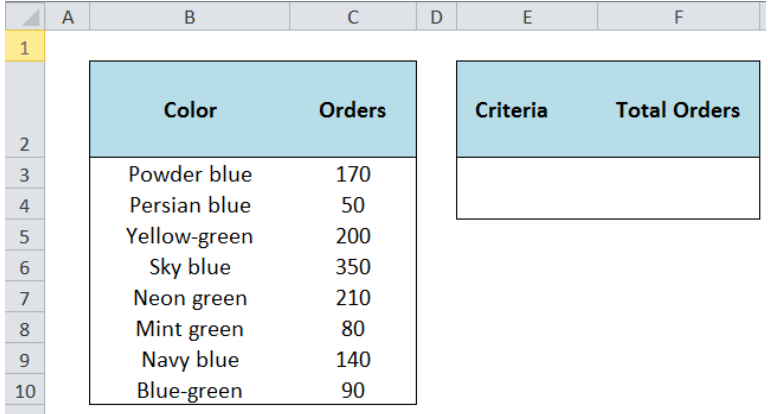Figure 2.  Sample data to sum cells based on specific text in another cell

## Sum Cells in Excel

In summing cells based on the text of other cells, we can use either SUMIF or SUMIFS.

## SUMIF function in Excel

SUMIF sums the values in a specified range, based on one given criteria

### Syntax

`=SUMIF(range,criteria, [sum_range])`

Where

• Range: the data range that will be evaluated using the criteria
• Criteria: the criteria or condition that determines which cells will be added
• Sum_range: the cells that will be added; if left blank, “sum_range” = “range” which means that the range of data that will be added is the same range of data evaluated

## SUMIFS function in Excel

Similar to SUMIF, SUMIFS also sums the values in a specified range, but it can be based on one or more specified criteria.

### Syntax

`=SUMIFS(sum_range, criteria_range1, criteria1,[criteria_range2],[criteria2]...)`

• Sum_range: the cells that will be added
• Criteria_range1: the data range that will be evaluated using the criteria
• Criteria1:  the criteria or condition that determines which cells will be added
• Criteria_range2 and criteria2 are optional; only applied when there are more criteria as specified

Sum If Color is Blue using SUMIF

In cell F3, enter the formula:

`=SUMIF(B3:B10,"*"&"Blue"&"*",C3:C10)`

This formula returns the sum of all orders in column C with any variant of color blue in column B.  As shown below,  the sum of 170, 50, 350, 140 and 90 is 800.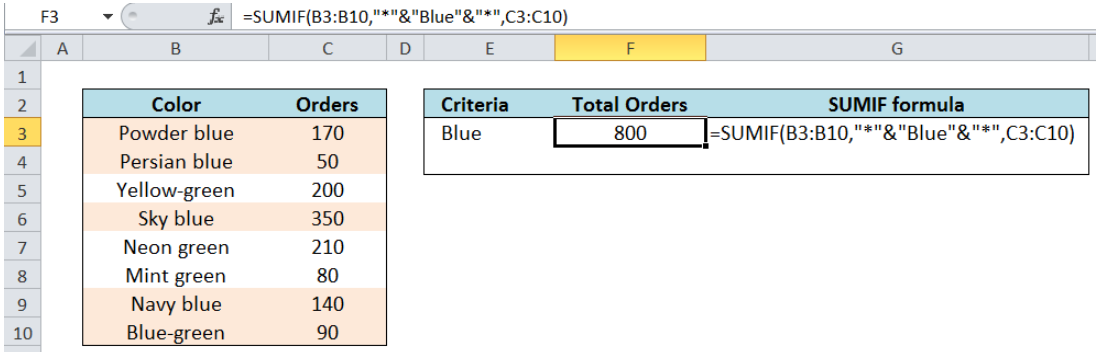Figure 3.  Entering the formula for SUMIF to sum orders of color Blue

### Important Notes:

• We want to add all orders in any variant of color blue.  Hence, our criteria should be *Blue* which includes all cells which may have texts before or after the word blue.
• This part of the formula: “*”&”Blue”&”*” means *Blue*
• The asterisk * is used as a wildcard in Excel, which means any number of characters
• The ampersand “&” is used to concatenate texts or strings

## Alternative Formula

In cell E4, enter the text string “ *Blue* ”.

In cell F4, enter the formula:

`=SUMIF(B3:B10,E4,C3:C10)`

This formula also sums all orders in column C that contains the text Blue in column B.  Putting asterisks in both sides of the text Blue and using *Blue* as the criteria simplifies the formula.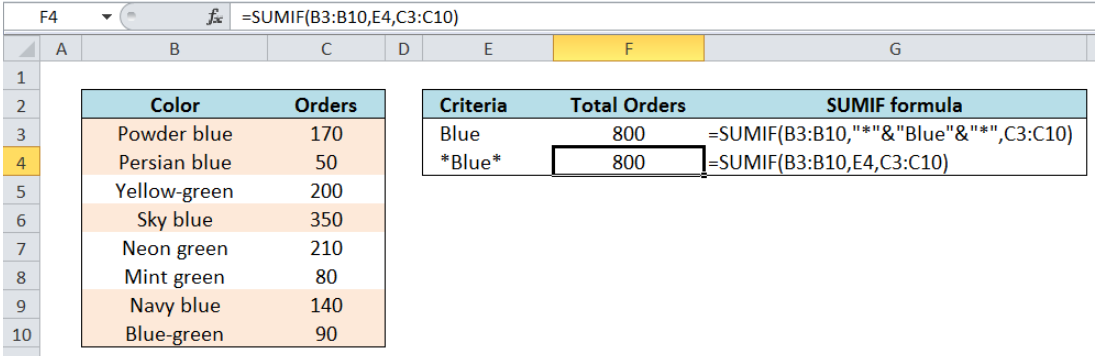Figure 4.  Simpler formula for SUMIF to sum orders of color Blue

Sum If Color is Blue using SUMIFS

In cell F3, enter the formula:

`=SUMIFS(C3:C10,B3:B10,"*"&"Blue"&"*")`

In cell F4, we use the alternative formula with reference to the criteria *Blue* in cell E9

`=SUMIFS(C3:C10,B3:B10,E9)`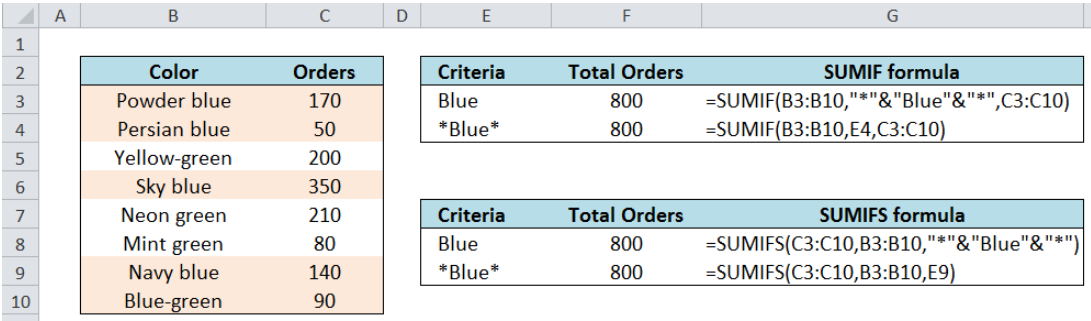Figure 5.  Comparison of SUMIF and SUMIFS in summing orders with color Blue

Both the SUMIF and SUMIFS functions return the same results. Just be mindful of the difference in syntax of these two functions. Remember, SUMIF can only be used if there is one criterion, while SUMIFS can be used with one or more criteria.

Most of the time, the problem you will need to solve will be more complex than a simple application of a formula or function. If you want to save hours of research and frustration, try our live Excelchat service! Our Excel Experts are available 24/7 to answer any Excel question you may have. We guarantee a connection within 30 seconds and a customized solution within 20 minutes.

### Did this post not answer your question? Get a solution from connecting with the expert.Another blog reader asked this question today on Excelchat:
Solution examplesamending a formula so that there is another criteria and if it does not meet this criteria then we stick with the original criteria
Solved by A. B. in 40 minsI am looking for a formula to SUM column L, if Column A matched the relevant date and column C matches the name
Solved by Z. Y. in 16 minsI am trying to utilize a complicated sumif or sumifs formula that can pull a total amount from a date and a drop down box from another tab.
Solved by E. B. in 15 minsI have a spreadsheet that totals invoices. A bunch of cells at the bottom of the spreadsheet calculates totals based on which department the invoice is charged to. I'd like to add a function where it also considers the date (so if it's an invoice in January, it goes to a January total, February, etc.). But I can't figure out how to write it properly...
Solved by K. J. in 27 minsIf I want to write a formula that states" "If this name shows up K24, and this word shows up in J24 pull this number from M24" I can creat that formula - however I need to drag this down so it pulls from row 24 - 60 - is there way to do this?
Solved by F. D. in 40 mins## Subscribe to Excelchat.coAnother blog reader asked this question today on Excelchat: